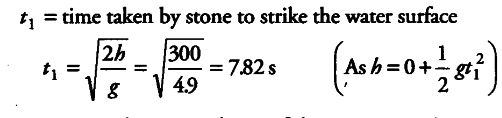# A stone dropped from the top of a tower of height 300 m

A stone dropped from the top of a tower of height 300 m in high splashes into the water of a pond near the base of the tower. When is the splash heard at the top given that the speed of sound in air is 340 m\${{s}^{-1}}\$? (5=9.8 m\${{s}^{-2}}\$)

Given, h = 300 m, g = 9.8 m/{{s}^{2}}, v = 340 m\${{s}^{-1}}\$t_{ 2 } = time taken to reach top of the tower
= h/v = 300/340 = 0.885
Total time t = time to hear splash of sound =
t_{ 1 } +t_{ 2 } =7.82 + 0.88 = 8.705